# LET function in EXCEL 365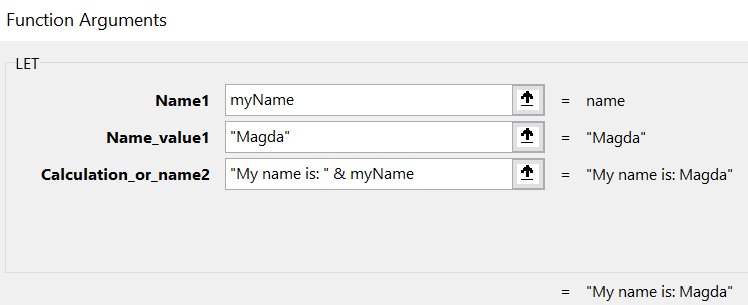Microsoft Excel 365 gives us a new function – LET(). It is not available for every Microsoft 365 subscriber though. A few weeks ago my Excel did not have this function, but now I can work with it. I did a few examples when I was learning this feature and want to share them with you today.

# What is LET function?

Microsoft’s definition:

The LET function assigns names to calculation results. This allows storing intermediate calculations, values, or defining names inside a formula. These names only apply within the scope of the LET function. Similar to variables in programming, LET is accomplished through Excel’s native formula syntax.

LET function description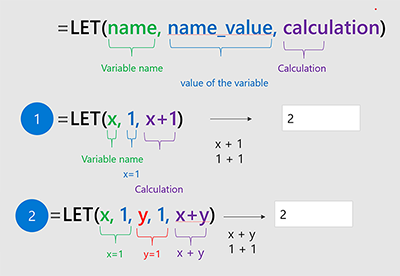Few more details about the parameters:

Name1 – a name of the variable/expression; has to start with a letter and cannot be the output of a formula

Name_value1..127 – a value/formula for the corresponding name

Calculation_or_name2..127 – this one has two modes: the first serves as a place for calculations using names defined before, and the second acts as Name1, i.e. defines a name for another variable in relation to Name_value_2..127

# Example 1 – single name

Here is the simplest example of the LET function application. We need to provide at least three parameters:

Name1 – the name starting with a letter of a single variable/expression; cannot use formulas here `myName`

Name_value1 – an actual value or an expression `CONCAT("M","a","g","d","a")`

Calculation_or_name2 – can be a formula that uses the Name1 `"My name is: " & myName`

As a result we will get a string `My name is: Magda`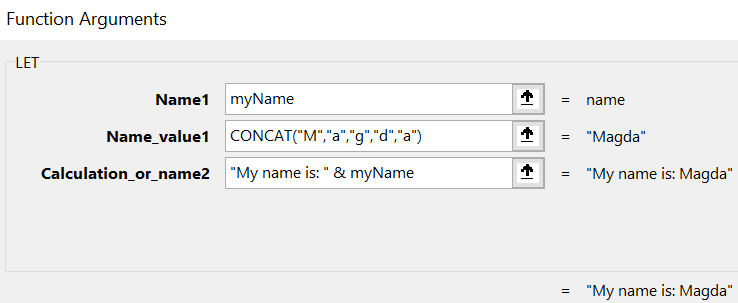# Example 2 – multiple names

The definition of the LET function allows us to provide more (up to 126) defined pairs (name:value), so let’s see how we can add one more.

Name1 – the name starting with a letter of a single variable/expression; cannot use formulas here `myName`

Name_value1 – an actual value or an expression `CONCAT("M","a","g","d","a")`

Calculation_or_name2 – this one changes now, as it becomes a name of a second variable, so all the rules apply `liveIn`

Name_value2 – an actual value or an expression of the second name `"Southampton"`

Calculation_or_name3 – now the final calculation is that uses both names the Name1 and Name2 `"My name is: " & myName & " and I live in: “&liveIn`

As a result, we will get a string `My name is: Magda and I live in: Southampton`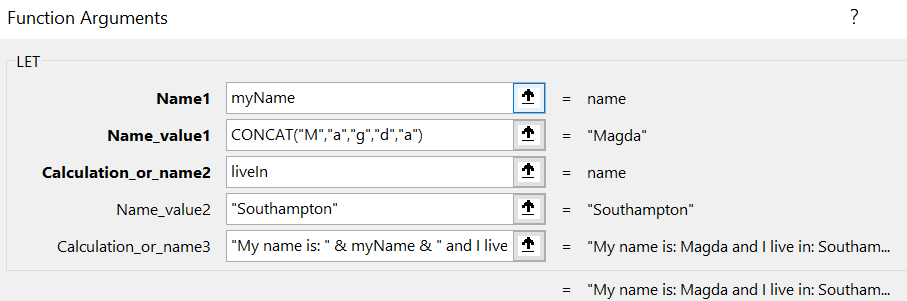# Example 3 – reusing values

As my last example I want to see the parameters being reused in multiple calculations

Name1 – the name starting with a letter of a single variable/expression; cannot use formulas here `myName`

Name_value1 – an actual value or an expression `CONCAT("M","a","g","d","a")`

Calculation_or_name2 – second variable `text`

Name_value2 – as a value using formula with Name1 `SUBSTITUTE(myName&" was here"," ","-")`

Calculation_or_name3 – in the final calculation I am using second variable text multiple times `UPPER(text)&" | "&LOWER(text)&" | "&PROPER(text)`

As a result, we will get a string `MAGDA-WAS-HERE | magda-was-here | Magda-Was-Here`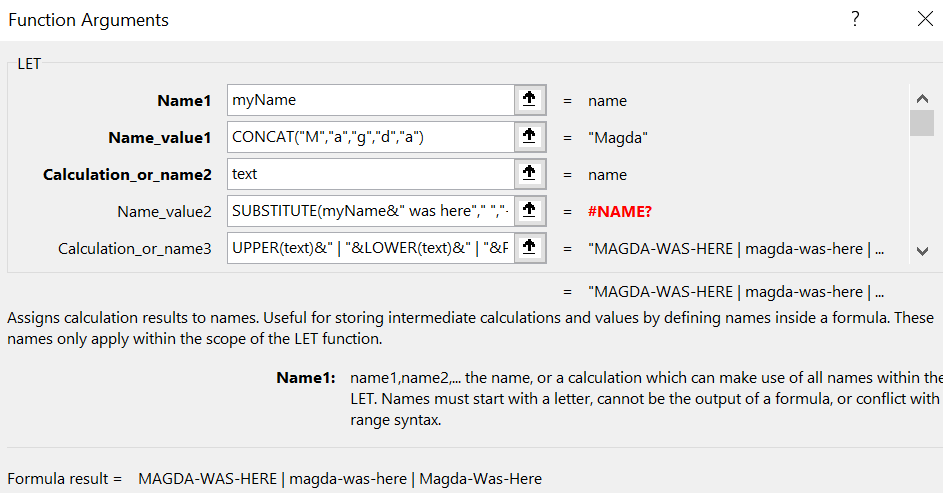So far I did not find why it is throwing an error #NAME?, but it is gone when I remove the first variable.

Thank you,

Magda

Tags:

Categories:

Updated: